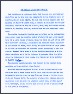Most text-books on Astronomy state that the sun is a middle-sized star. This may be true when one compares it to the relative sizes of super-giants and white dwarfs. But how does the sun compare with the majority of stars? To answer this, I decided to calculate the stellar luminosity function as well as I could, using only the limited facilities at my disposal, that is, a slide rule and the Observers' Handbook.

The stellar luminosity function may be defined as the mathematical curve which shows the relative number of stars for each given magnitude for a given volume of space. Using the table on p.87 of the 1968 Observers' Handbook, I calculated the absolute visual magnitude of the nearest 55 stars, which occupy the space within 16 light years of the sun. To calculate the absolute visual magnitudes, I used the formula M=m+5 log r where M is the absolute magnitude, m is the visual magnitude and r is the distance in parsecs. Since the table gives the distance in light years, the formula had to be modified to M=m+5-5 log (D/3.26).

The absolute magnitudes were then rounded to the nearest whole magnitude and then plotted. (See fig.1.)

The sun's absolute magnitude is 4.8. It can be seen readily that there are only 3 stars within the 16 light year radius that are intrinsically brighter than the sun. They are Sirius A (1.45), Altair (2.3) and Procyon (2.7). The majority of stars fall between absolute magnitudes 9 and 16, with the greatest number being of 13th magnitude. Therefore, it may be seen that the sun is not a typical star in this part of the galaxy.

The curve descends more sharply than it ascends. This may be caused by evolutionary factors and may throw light on the density of interstellar gases in this area during the formation of the galaxy.

The fact that the sun is brighter than its neighbours may be seen fro the list of luminosities on the same page, but I feel that a survey of the absolute magnitudes may be more readily understood.

K.E. Chilton
Hamilton Centre

File:
AttachmentSizeThe Stellar Luminosity Function (PDF)240.83 KBThe Stellar Luminosity Function (DJVU)502.75 KB
Year:
1968
Pages:
3
ArchiveTags: